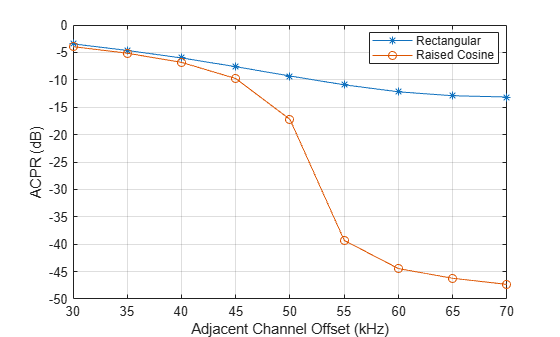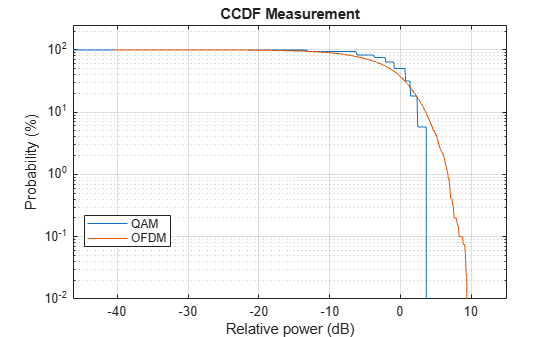Main Content

## ACPR and CCDF Measurements with MATLAB System Objects

### ACPR Measurements

This example shows how to measure the adjacent channel power ratio (ACPR) from a baseband, 50 kbps QPSK signal. ACPR is the ratio of signal power measured in an adjacent frequency band to the power from the same signal measured in its main band. The number of samples per symbol is set to four.

Set the samples per symbol (`sps`) and channel bandwidth (`bw`) parameters.

```sps = 4; bw = 50e3;```

Generate 10,000 4-ary symbols for QSPK modulation.

`data = randi([0 3],10000,1);`

Construct a QPSK modulator and then modulate the input data.

```qpskMod = comm.QPSKModulator; x = qpskMod(data);```

Apply rectangular pulse shaping to the modulated signal. This type of pulse shaping is typically not done in practical system but is used here for illustrative purposes.

`y = rectpulse(x,sps);`

Construct an ACPR System object. The sample rate is the bandwidth multiplied by the number of samples per symbol. The main channel is assumed to be at 0 while the adjacent channel offset is set to 50 kHz (identical to the bandwidth of the main channel). Likewise, the measurement bandwidth of the adjacent channel is set to be the same as the main channel. Lately, enable the main and adjacent channel power output ports.

```acpr = comm.ACPR('SampleRate',bw*sps,... 'MainChannelFrequency',0,... 'MainMeasurementBandwidth',bw,... 'AdjacentChannelOffset',50e3,... 'AdjacentMeasurementBandwidth',bw,... 'MainChannelPowerOutputPort', true,... 'AdjacentChannelPowerOutputPort',true);```

Measure the ACPR, the main channel power, and the adjacent channel power of signal y.

`[ACPRout,mainPower,adjPower] = acpr(y)`
```ACPRout = -9.3071 ```
```mainPower = 28.9389 ```
```adjPower = 19.6318 ```

Change the frequency offset to 75 kHz and determine the ACPR. Since the AdjacentChannelOffset property is nontunable, you must first release `acpr`. Observe that the ACPR improves when the channel offset is increased.

```release(acpr) acpr.AdjacentChannelOffset = 75e3; ACPRout = acpr(y)```
```ACPRout = -13.1702 ```

Release `acpr` and specify a 50 kHz adjacent channel offset.

```release(acpr) acpr.AdjacentChannelOffset = 50e3;```

Create a raised cosine filter and filter the modulated signal.

```txfilter = comm.RaisedCosineTransmitFilter('OutputSamplesPerSymbol', sps); z = txfilter(x);```

Measure the ACPR for the filtered signal, z . You can see that the ACPR improves from -9.5 dB to -17.7 dB when raised cosine pulses are used.

`ACPRout = acpr(z)`
```ACPRout = -17.2245 ```

Plot the adjacent channel power ratios for a range of adjacent channel offsets. Set the channel offsets to range from 30 kHz to 70 kHz in 10 kHz steps. Recall that you must first release `hACPR` to change the offset.

```freqOffset = 1e3*(30:5:70); release(acpr) acpr.AdjacentChannelOffset = freqOffset;```

Determine the ACPR values for the signals with rectangular and raised cosine pulse shapes.

```ACPR1 = acpr(y); ACPR2 = acpr(z);```

Plot the adjacent channel power ratios.

```plot(freqOffset/1000,ACPR1,'*-',freqOffset/1000, ACPR2,'o-') xlabel('Adjacent Channel Offset (kHz)') ylabel('ACPR (dB)') legend('Rectangular','Raised Cosine','location','best') grid```### CCDF Measurements

This example shows how to use the Complementary Cumulative Distribution Function (CCDF) System object to measure the probability of a signal's instantaneous power being greater than a specified level over its average power. Construct the `comm.CCDF` object, enable the PAPR output port, and set the maximum signal power limit to 50 dBm.

`ccdf = comm.CCDF('PAPROutputPort',true,'MaximumPowerLimit', 50);`

Create an OFDM modulator having an FFT length of 256 and a cyclic prefix length of 32.

`ofdmMod = comm.OFDMModulator('FFTLength',256,'CyclicPrefixLength',32);`

Determine the input and output sizes of the OFDM modulator object using the `info` function of the `comm.OFDMModulator` object.

`ofdmDims = info(ofdmMod)`
```ofdmDims = struct with fields: DataInputSize: [245 1] OutputSize: [288 1] ```
```ofdmInputSize = ofdmDims.DataInputSize; ofdmOutputSize = ofdmDims.OutputSize;```

Set the number of OFDM frames.

`numFrames = 20;`

Allocate memory for the signal arrays.

```qamSig = repmat(zeros(ofdmInputSize),numFrames,1); ofdmSig = repmat(zeros(ofdmOutputSize),numFrames,1);```

Generate the 64-QAM and OFDM signals for evaluation.

```for k = 1:numFrames % Generate random data symbols data = randi([0 63],ofdmInputSize); % Apply 64-QAM modulation tmpQAM = qammod(data,64); % Apply OFDM modulation to the QAM-modulated signal tmpOFDM = ofdmMod(tmpQAM); % Save the signal data qamSig((1:ofdmInputSize)+(k-1)*ofdmInputSize(1)) = tmpQAM; ofdmSig((1:ofdmOutputSize)+(k-1)*ofdmOutputSize(1)) = tmpOFDM; end```

Determine the average signal power, the peak signal power, and the PAPR ratios for the two signals. The two signals being evaluated must be the same length so the first 4000 symbols are evaluated.

`[Fy,Fx,PAPR] = ccdf([qamSig(1:4000),ofdmSig(1:4000)]);`

Plot the CCDF data. Observe that the likelihood of the power of the OFDM modulated signal being more than 3 dB above its average power level is much higher than for the QAM modulated signal.

```plot(ccdf) legend('QAM','OFDM','location','best')```Compare the PAPR values for the QAM modulated and OFDM modulated signals.

```fprintf('\nPAPR for 64-QAM = %5.2f dB\nPAPR for OFDM = %5.2f dB\n',... PAPR(1), PAPR(2))```
```PAPR for 64-QAM = 3.65 dB PAPR for OFDM = 9.44 dB ```

You can see that by applying OFDM modulation to a 64-QAM modulated signal, the PAPR increases by 5.8 dB. This means that if 30 dBm transmit power is needed to close a 64-QAM link, the power amplifier needs to have a maximum power of 33.7 dBm to ensure linear operation. If the same signal were then OFDM modulated, a 39.5 dBm power amplifier is required.

## Support

#### Bridging Wireless Communications Design and Testing with MATLAB

Download white paper